index: click on a letter A B C D E F G H I J K L M N O P Q R S T U V W X Y Z A to Z index index: subject areas numbers & symbols sets, logic, proofs geometry algebra trigonometry advanced algebra & pre-calculus calculus advanced topics probability & statistics real world applications multimedia entrieswww.mathwords.com about mathwords website feedback

Rationalizing the Denominator

The process by which a fraction is rewritten so that the denominator contains only rational numbers. A variety of techniques for rationalizing the denominator are demonstrated below.

 Square Roots (a > 0, b > 0, c > 0) Examples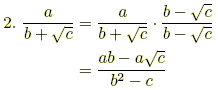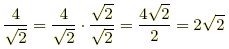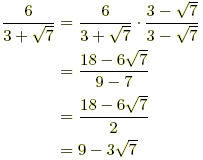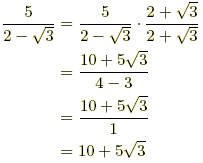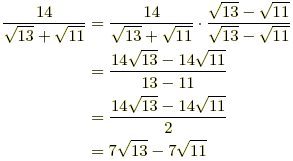nth Roots (a > 0, b > 0, c > 0) Examples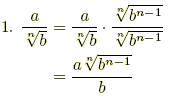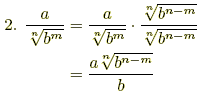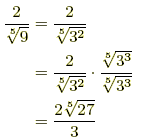Example